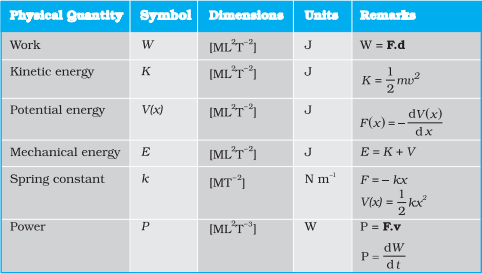During a collision between two bodies if there is a loss of kinetic energy, then the collision is said to be an inelastic collision.

In inelastic collision, the linear momentum is conserved but the energy is not conserved. The loss of kinetic energy usually results in the form of heat or sound energy.

If two bodies stick together, after colliding, the collision is perfectly inelastic but it is a special case of inelastic collision called plastic collision. (e.g.) a bullet striking a block of wood and being embedded in it.

Let us consider a simple situation in which the inelastic head on collision between two bodies of masses mA and mB takes place. Let the colliding bodies be initially moved with velocities u1 and u2. After collision, both bodies stick together and move with common velocity v.

Total momentum of the system before collision = mAu1 + mBu2

Total momentum of the system after collision = mass of the composite body?

common velocity = (mA + mB) v

By law of conservation of momentumThus, knowing the masses of the two bodies and their velocities before collision, the common velocity of the system after collision can be calculated.

If the second particle is initially at rest i.e. u2 = 0 then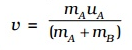Kinetic energy of the system before collision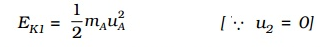And kinetic energy of the system after collision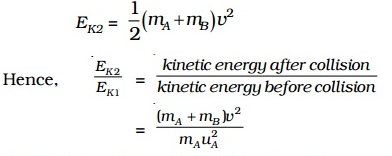Substituting the value of v in the above equation,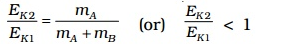It is clear from the above equation that in a perfectly inelastic collision, the kinetic energy after impact is less than the kinetic energy before impact. The loss in kinetic energy may appear as heat energy.